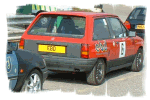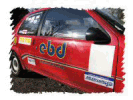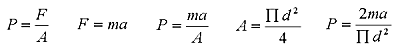ebd Blinded By Science..... - well at least maybe when I've finished writing it!
Just a start!!! - Perhaps Matt will stop getting upset for a bit now!

<Where:

F = Force, (Newtons, N)
M = Reciprocating Mass (Kg)
θ = Crank Angle (0 @ TDC)
L = Conrod Length (m)
N = engine rpm

If R/L > 0.3 Then it is accurate enough to use 1st 2 Terms

CSA Of Rod = 1.075391x10-4 m2

Mass of Piston:
OEM = 395g = 0.395kg
Wosner =  315g = 0.315kg
Small End Mass:
ebd Modified Rods = 135.5g = 0.1355kg
Total Reciprocating Mass:
Original = 0.5305kg
ebd modified = 0.4505kg

Max designed for rpm = 8500rpm

Stroke = 77mm = 0.077m

Bore = 76mm = 0.076m

Rod Length = 133.5mm = 0.1335m

Piston Speed (average)
Piston Speed (Maximum)

Since we have the maximum acceleration of the piston & therefore the piston @ max rpm we can calculate the stress on the conrod.  Also note that this shows that well designed rods do not fail under compression

Where:

Rod material Tensile strength = 430 N/mm2 (MPa )

Given max stress on

Since we have the maximum acceleration of the piston & therefore the piston @ max rpm we can calculate the stress on the conrod.  Also note that this shows that well designed rods do not fail under compression

Where: